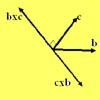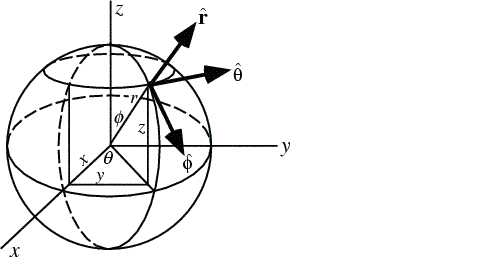#### You may also likeA quadrilateral changes shape with the edge lengths constant. Show the scalar product of the diagonals is constant. If the diagonals are perpendicular in one position are they always perpendicular?As a quadrilateral Q is deformed (keeping the edge lengths constnt) the diagonals and the angle X between them change. Prove that the area of Q is proportional to tanX.### Multiplication of Vectors

An account of multiplication of vectors, both scalar products and vector products.

# Air Routes

##### Age 16 to 18 Challenge Level:

The solution here uses scalar products of vectors. For a similar calculation using the cosine rule instead of scalar products see the problem Flight Path.

Good solutions were sent in by Thomas Lauffenberger, Angus Balkham of Bexhill College and Andrei Lazanu of School No. 205, Bucharest, Romania.

Here is Andrei's solution:

Two things are to be observed for solving this problem:

1) the angle $\alpha$ between two vectors ${\bf a}$ and ${\bf b}$ can be determined from their scalar product as follows: $$\cos \alpha = {{\bf a}.{\bf b}\over ab}$$ where a and b are the moduli of the vectors.

2) On Earth approximated with a sphere, if one knows the latitude and longitude of a point (city), the vectors with the origin in the centre of the Earth and ending in the respective city are completely determined.

The position on a sphere is usually described using the spherical coordinates: - the azimuthal angle, the angle in the xOy plane, and, the polar angle, from the z axis, as illustrated below:On the sphere $r$ is fixed, and I'll first work on the unit sphere. For the problem, the azimuthal angle gives the longitude, while the polar angle is ($90^o$ - latitude).

The relation between the spherical coordinates and the Cartesian ones on the unit sphere is: $$x=\cos \theta \sin \phi,\ y = \sin \theta \sin \phi,\ z = \cos \phi.$$ For London, angle $\theta$ is ($90^o$ - $52^o$), i.e. $38^0$ and angle $\phi$ is 0°. So, its coordinates are: $$x_1 = \sin 38^o \cos 0^o = \sin 38^o,\ y_1 = \sin 38^o \sin 0^o = 0,\ z_1 = \cos 38^o.$$ For Cape Town angle $\phi$ is $90^o + 34^o = 124^o$ and angle $\theta$ is $18^o$. Its coordinates are: $$x_2 = \sin 56^o \cos 18^o,\ y_2 = \sin 56^o \sin 18^o,\ z_2 = \cos 124^o = -\cos 56^o.$$

The scalar product of the two vectors characterised by their Cartesian coordinates: $\vec{OA}=x_1{\bf i}+y_1{\bf j} + z_1{\bf k}$ and $\vec{OB}=x_2{\bf i}+y_2{\bf j} + z_2{\bf k}$ is $$\vec{OA}\cdot \vec{OB}= x_1x_2+y_1y_2+z_1z_2.$$ The product of the vectors corresponding to London and Cape Town is: $$\vec{OL}\cdot \vec{OC}= \sin 38^o \sin 56^o \cos 18^o - \cos 38^o \cos 56^o = 0.0447754018.$$

So, if angle $LOC$ has its cosine value 0.0447754018, then its measure is $87.43370046^o$.

Each two points of a sphere are on a big circle of the sphere, having as centre the centre of the sphere. The angle between these points is the angle determined above.

The circumference of the Earth (considering it is a sphere) is: $2\pi \times 6367$ km

This value corresponds to $360^o$. So, to the angle $87.43370046^o$ corresponds the following distance in kilometres: $${87.43370046 \over 360} \times 2\pi \times 6367 = 9716.079$$ which we round to 9716 km.

I looked in Encarta and I found that the distance between the two cities is 9689 km, so the approximation is very good.

The distance traveled by the plane is a circular arc, with radius 6373 km. Applying a similar procedure, I observe that the plane traveled 9725.2359 km.

The formula for obtaining the speed is v = d/t, where d is the distance and t - the time. So, the average speed is 884 km/h (to 3 significant figures), a value that is again reasonable.